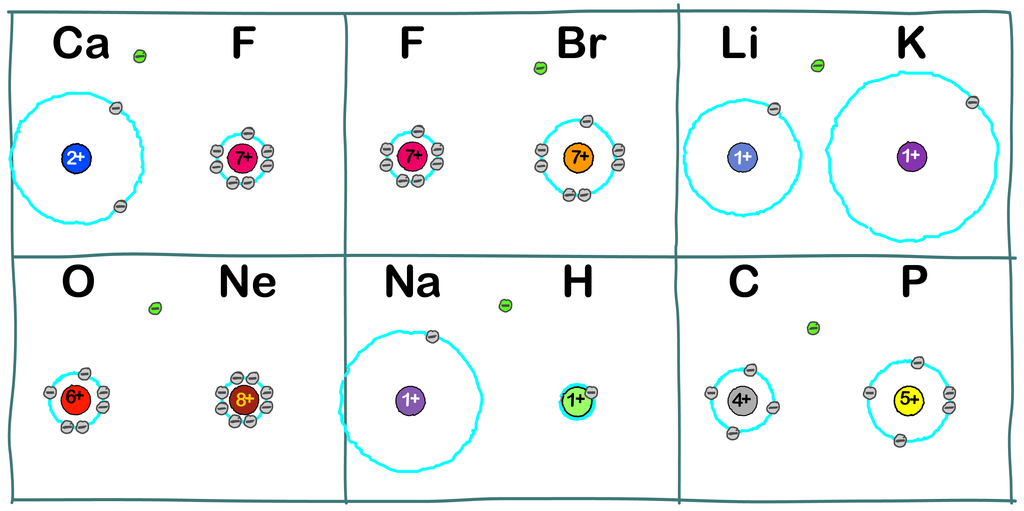# 2 - Predict and Explain Electron Attraction

## Where Will The Electron End Up?

The diagram below has six exercises. Each exercise consists of two atoms, plus one additional electron. Your task is to decide which atom has the strongest claim on the free electron.

### You're the teacher - you do it first!

1. Examine each exercise.
2. Compare the core charge, radius and valence configuration of each pair of atoms.
3. Decide which atom has the strongest attraction for the free electron.
4. Draw an arrow from the electron's starting point to the valence shell vacancy where it comes to rest.### Now It's Your Students' Turn.2. Print it, either colour or BW, and distribute it to your students.
3. Let them read the instructions on the pdf file.
4. See how your students perform!

I'm confident that they can intuitively do what students using traditional methods could do only after several days of instruction.

#### 1. Calcium and Fluorine

No question. The electron can get closer to a stronger core charge if it goes to fluorine

#### 2. Fluorine and Bromine

The 7+ core charges are equally attractive to the electron, so radius is the deciding factor. The electron can get closer to the 7+ core charge if it goes into an empty valence position in fluorine.#### 3. Lithium and Potassium

Once again, since the core charges are identical, the electron goes to the smaller atom, lithium. While bonding situations like this are rare, the relative reactivity of lithium and potassium can be predicted using the same reasoning.

#### 4. Oxygen and Neon

Students will have to consider this one for a few extra seconds. Neon has the stronger core charge and the smaller radius. But neon's valence shell is full, and cannot accept any more electrons. So - oxygen must be the winner.

#### 5. Sodium and Hydrogen

Same group, same core charge, so why does hydrogen win the electron? Because hydrogen's radius is so small. As a chemistry teacher, can you see why the reaction between sodium and water produces hydrogen gas?

#### 6. Carbon and Phosphorus

While all of the other cases probably produced nearly unanimous results, this one is a puzzler. Your students won't be unanimous on this one. Phosphorus has the greater core charge, so phosphorus should win. But carbon has the smaller radius, so carbon should win.

### The Colors Of The Ross Table

Here we reach the honest limits of using the diagrams alone to decide these questions. To resolve question 6, we must go to lesson 3.

Lesson 1 - Learn the three salient features of each atom, and follow how they change across the periodic table.

Lesson 2 - Learn how students can make accurate predictions, with nearly no instruction!

Lesson 3 - Take a closer look at the elements of the second row.

Lesson 4 - Origins of the Ross model of the atom

Lesson 5 - Covalent bonding

Lesson 6 - Ionic Bonding

Lesson 7 - A post-modern model of science.

Lesson 8 - Learning with IntuitivScience.